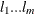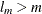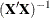The PDLREG Procedure

ODS Graphics

Statistical procedures use ODS Graphics to create graphs as part of their output. ODS Graphics is described in detail in Chapter 21: Statistical Graphics Using ODS in SAS/STAT 13.2 User's Guide.

Before you create graphs, ODS Graphics must be enabled (for example, with the ODS GRAPHICS ON statement). For more information about enabling and disabling ODS Graphics, see the section "Enabling and Disabling ODS Graphics" in that chapter.

The overall appearance of graphs is controlled by ODS styles. Styles and other aspects of using ODS Graphics are discussed in the section "A Primer on ODS Statistical Graphics" in that chapter.

PROC PDLREG assigns a name to each table it creates. You can use these names to reference the table when using the Output Delivery System (ODS) to select tables and create output data sets. These names are listed in the following table.

Table 21.2: ODS Tables Produced in PROC PDLREG

ODS Table Name

Description

Option

ODS Tables Created by the MODEL Statement

ARParameterEstimates

Estimates of autoregressive parameters

NLAG=

CholeskyFactor

Cholesky root of gamma

NLAG= and ALL

Coefficients

Coefficients for first NLAG observations

NLAG= and (COEF or ALL)

ConvergenceStatus

Convergence status table

default

CorrB

Correlation of parameter estimates

CORRB

CorrGraph

Estimates of autocorrelations

NLAG=

CovB

Covariance of parameter estimates

COVB

DependenceEquations

Linear dependence equation

Dependent

Dependent variable

default

DWTest

Durbin-Watson statistics

DW=

DWTestProb

Durbin-Watson statistics and

DW=

p-values

DWPROB

ExpAutocorr

Expected autocorrelations

{NLAG= and (COEF or ALL)} or {NLAG=() where}

FitSummary

Summary of regression

default

GammaInverse

Gamma inverse

NLAG= and (GINV or ALL)

IterHistory

Iteration history

ITPRINT

LagDist

Lag distribution

default

ParameterEstimates

Parameter estimates

default

ParameterEstimatesGivenAR

Parameter estimates assuming AR parameters are given

NLAG=

PartialAutoCorr

Partial autocorrelation

PARTIAL

PreMSE

Preliminary MSE

NLAG=

XPXIMatrixmatrix

XPX

XPXMatrixmatrix

XPX